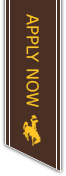# R commands that we use in Methods

### Basic Stuff

Control-Enter
Take a command from the script and run it (for windows)
Note that it's control-R for regular R (not R Studio)

Command-Enter
Take a command from the script and run it (for MAC)
Note that it's command-R for regular R (not R Studio)

?plot
Read the help file on plot

??plot
Search for all commands that have plot in the description

#Remember to fix this
A comment that won't be run if you do control-r

x <- 2
Assign the value of 2 to the box named x

x <- c(2,4,3,5,7)
Assign the vector of numbers 2 4 3 5 and 7 to the box named x

x <- "hello"
Assign the characters hello to the box named x

ls()
See all the variables (boxes) that you've created

rm(x)
Remove the box named x from your list of variables

Control-L
If you press control and l you'll clear the console (commands you've run)

### Data manipulation

Read in the data set from the url, and save in a box called x with the header names

Read in the data when it's tab delimited (verses comma delimited)

Read in the data but skip the first 3 lines (of text)

x[2,]
In the data set x, only use row 2

x[,3]
In the data set x, only use column 3

x[2,3]
In the data set x grab the value in row 2 column 3

See just first six rows of the dataset x

nrow(x)
The number of rows in dataset x

round(x,5)
round the number x to 5 decimal places

y <- x[x\$category=="red",]
In data set x, find only the rows where the categorical value is red and store that in a box called y

x<-rnorm(100,3,2)
Create 100 random numbers that are normal with a mean of 3 and sd 2. Store it in x

## Fixing Errors in the data

dataset\$nums <- as.numeric(as.character(dataset\$nums))
Turn factors (through characters) into numbers
Use this when numbers are being used like categories

x <- x[x\$variable>0,]
In data set x use only the rows where the variable is greater than zero
Use this to remove zeros and negatives

x <- x[x\$variable>=0,]
In data set x use only the rows where the variable is zero or greater
Use this to remove negatives

x <- x[x\$variable < 999,]
In data set x use only the rows where the variable is less than 999
Use this to fix large outliers

x <- na.omit(x)
The dataset x except remove any rows that have an "NA" value
Use this when you have NA's in the dataset

x\$category[x\$category=="A"] <- "B"
For the categorical variable change all the A's to be B
Use this when someone messes up the spelling or wording of a category

levels(x\$category) <- c("B", "B", "C", "D", "E")
To lump category A into category B
Use this when you want to get rid of category B (or when A and B are the same)

x\$variable[x\$variable<0] <- x\$variable[x\$variable<0]*-1
To change a negative value into a positive value
Use this when you want to alter specific values in a dataset

### Descriptive Statistics

min(x)
Find the minimum value in x

max(x)
Find the maximum value in x

sum(x)
the sum of x

mean(x)
the mean of x

sd(x)
the standard deviation of x

t.test(x)
A one sample test of mu=0, also confidence interval for the mean

t.test(x,y)
Two sample test of mu1=mu2, also confidence interval for the difference

t.test(x,y,paired=TRUE)
Two sample matched pairs t-test (with confidence interval)

### Plots

boxplot(x)
Make a boxplot of x

hist(x)
Draw a histogram of x

plot(x)
Plot the values of x in order (not actually that useful in this class)

plot(y~x)
Draw a scatterplot of y based on x

plot(y~x,xlim=c(0,100))
Plot y on x, but make the x axis go from 0 to 100

plot(y~x,ylim=c(0,100))
Plot y on x, but make the y axis go from 0 to 100

plot(y~x,col="red")
Plot y on x with red dots

plot(y~x,xlab="Time")
Plot y on x and label the x axis Time

plot(y~x,ylab="Height")
Plot y on x and label the y axis Height

plot(y~x,main="Height based on Time")
Plot y on x and write Height based on Time at the top

lines(y~x)
Add the line for y on x on top of whatever plot is already there

points(y~x)
Add the dots for y on x on top of whatever plot is already there

legend("topright",col=c("red","yellow","blue"),legend=c("high","medium","low"),lty=1)
Put a legend in the top right corner. Have the red line say high, etc.

par(mfrow=c(2,2))
Start putting 4 plots (2 rows, 2 columns) on one picture

x<-seq(0,10,length=1000)
y<-5+2*x
plot(y~x,type="l")
Plot the line y=5+2*x

### Regression

fit<-lm(y~x,data=flowers)
fit<-lm(flowers\$y~flowers\$x)
Predict y based on x, and save the results in a variable (box) called fit

plot(fit)
plot the residuals (4 different plots)

summary(fit)
Get slopes, p-values, R^2, and the standard error

confint(fit)
Computes condidence intervals for one or more parameters in an lm model called fit

lm(y~I(x^2),data=flowers)
Predict y based on x squared

lm(y~x+I(x^2)+q+w+q*w,data=flowers)
predict y based on x, x^2, q, w, and their interaction

plot(fit\$residuals~x)
Plot the residuals against x

predict.lm(fit,newdata=data.frame(x=10,q=2,w=5))
Used to make predictions

log(2)
log uses base e
log10(2)
log10 uses base 10
exp(2)
exponent on e
lm(y~log(x),data=flowers)
log does not use the I() notation1000 E. University Ave. Laramie, WY 82071
Accreditation | Virtual Tour | Emergency Preparedness | Employment at UW | Privacy Policy | Harassment & Discrimination | Accessibility